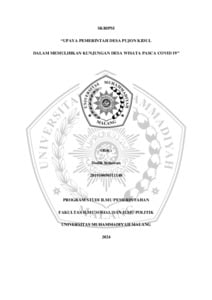## PENGARUH LEVERAGE DAN RASIO LIKUIDITAS TERHADAP EARNING PER SHARE (Studi Pada Perusahaan Yang Tergabung Dalam Indeks LQ45)

PURWONO, EKOADI (2009) PENGARUH LEVERAGE DAN RASIO LIKUIDITAS TERHADAP EARNING PER SHARE (Studi Pada Perusahaan Yang Tergabung Dalam Indeks LQ45). Other thesis, University of Muhammadiyah Malang.Preview
Text

## Abstract

Title : "Influence Leverage And Liquidity Ratio To Earning Per Share (Study At Company Merged Into By Index LQ45)". (Ekoadi Purwono, Drs. Dhaniel Syam MM, Ak, Dra. Siti Zubaidah MM, Ak). Purpose of research is to know how big influence leverage and liquidity ratio to earning per share. Analyzer applied is degree of operating leverage (DOL) degree financial of of leverage (DFL), Current Ratio, and Quick ratio. Measuring rod to determine how big influence leverage and liquidity ratio to earning per share was if level of signifikansi < 0,05. If signifikansi every variable < 0,05, hence there is influence signifikan to earning per share. leverage (DOL), degree financial of leverage (DFL), Current Ratio, and Quick ratio joinly/simultan influential to earning per share shown with level signifikansi 0,008. This thing means joinly/simultan there is significant influence to earning per share. While tee test result, variable degree of operating leverage (DOL), degree financial of of leverage (DFL), Current Ratio, and Quick ratio parsially doesn't have an effect on signifikan, but variable degree financial of leverage (DFL) which very influential to earning per share with level signifikansi 0,002 smaller than 0,05. Variable that is very influential to earning per share is degree financial of leverage (DFL), this thing is shown with biggest regression coefficient between all variables that is 0,410. From result of F test analysis and tee test, joinly fourth variable to influential to earning per share. While parsially doesn't have an effect on signifikan. Thereby first hypothesis in this proven research, but unprovable second hypothesis.

Item Type: Thesis (Other) H Social Sciences > HB Economic Theory Faculty of Economics > Department of Accounting (62201) Anggit Aldila 25 Jun 2012 08:38 25 Jun 2012 08:38 http://eprints.umm.ac.id/id/eprint/9450View Item